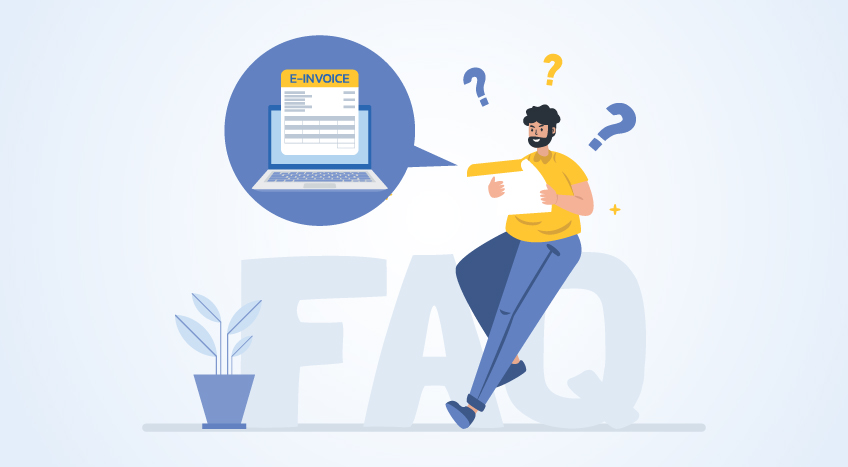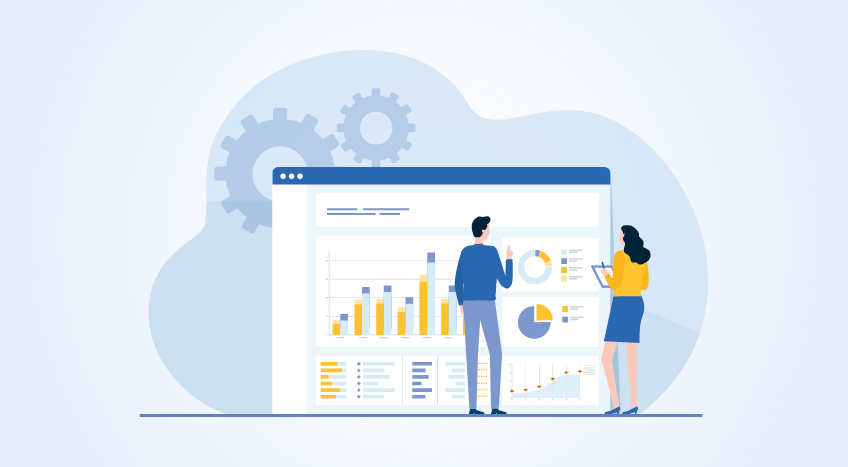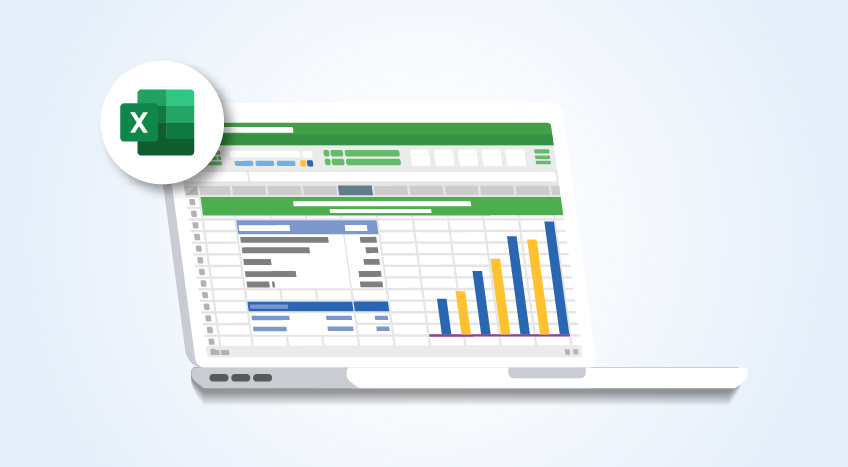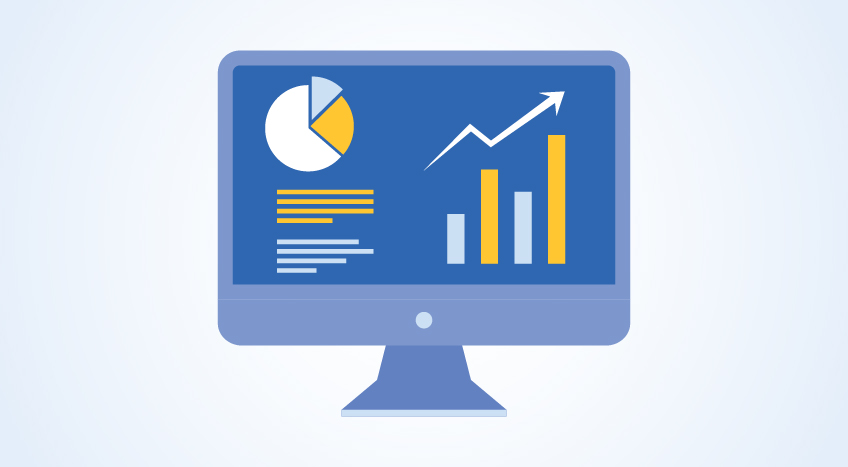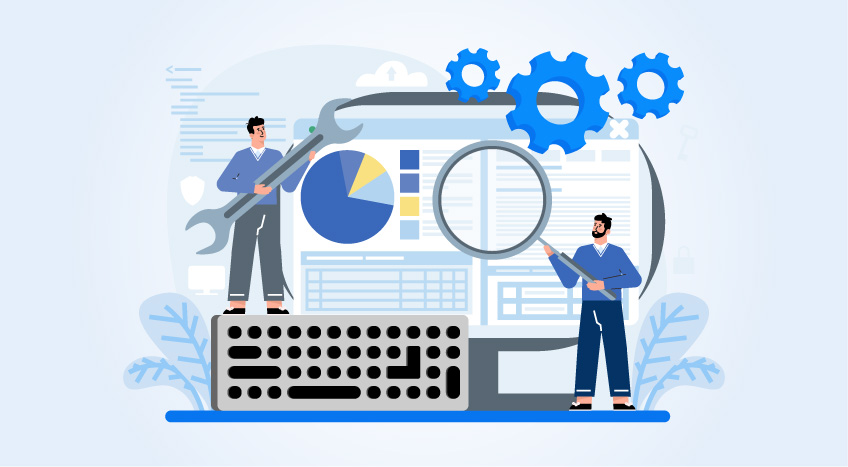# Accumulated Depreciation – Meaning & How it Works & Calculation

## What is Accumulated Depreciation

Accumulated depreciation is the total amount a company depreciates its assets, while depreciation expense is the amount a company's assets are depreciated for a single period. Essentially, accumulated depreciation is the total amount of a company's cost that has been allocated to depreciation expense since the asset was put into use.

## Depreciable Assets

Depreciable assets are those assets which fulfil the following conditions:

• It is expected to be used for more than one accounting period
• Have a limited useful life
• These are held for the purpose of production of goods and services and not for sale in the ordinary course of business

Depreciation Accounting covers all the assets, except for the following: -

• Forests, plantation and other similar regenerative natural resources
• Wasting assets
• Expenditure on research and development
• Goodwill
• Livestock
• Land

## How to Calculate Accumulated Depreciation?

To calculate accumulated depreciation, there are 3 important factors you need to consider.Now that you the 3 factors to consider the accumulated depreciation, you can calculate using the below formal.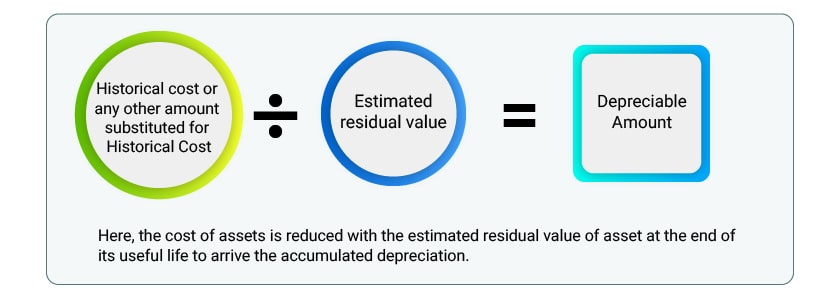Here, the cost of assets is reduced with the estimated residual value of an asset at the end of its useful life to arrive the accumulated depreciation.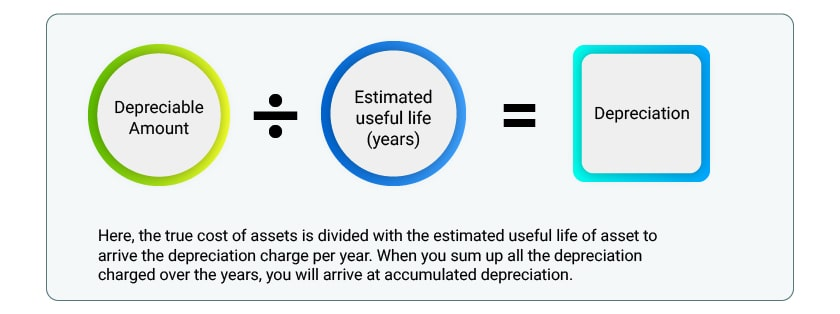## Accumulated Depreciation Examples

Machinery is purchased for Rs. 1,70,000 /- and at the end of the useful life of the machinery, the residual value is estimated at Rs. 70,000 /-. As per the estimation made by the company, the machinery will work for 5 years.

Considering the above example, the sum of expense to be allocated as depreciation in the accounting periods will be calculated as below:

 Acquisition Cost                 = Rs. 1,70,000.                      Less - Residual Value         = Rs.  70,000.                             Depreciable Amount           = Rs. 1,00,000.                             Estimated life of the asset = 5 Years.   Depreciaton= Depreciable amount / Useful  life of an asset                                                       =   Rs.  1,00,000 / 5                                                       =    Rs.  20,000 Per year

The Accumulated Depreciation of the machinery at the end of 5 years, assuming straight-line method of deprecation is Rs. 1,00,000 /- (Rs. 20,000 * 5)

## Features and Causes of Accumulated Depreciation

### Features of Depreciation

• Depreciation is a part of operating cost.
• It is a reduction in the value of an asset.
• The decrease in the value of an asset is gradual and continuous.

### Causes of Depreciation

• Physical wear and tear
• Physical deterioration
• Expiry of legal rights
• Obsolescence

## Methods of Accumulated Depreciation

Basically, methods for providing depreciation are based on the formula, developed on a study of the behaviour of the assets over a period of years. This is done for readily computing the amount of depreciation suffered by different forms of assets. However, these methods should be applied only after carefully considering the nature of the asset and the conditions under which it is being used.

 Methods Explanation Straight-line method of Depreciation According to this method, an equal amount is written off every year during the working life of an asset so as to reduce the cost of the asset to its residual or nil value at the end of its useful life. SLM method results in a constant charge over the useful life if the residual value of the asset does not change Written down value or diminishing balance method Under this system, a fixed percentage of the diminishing value of the asset is written off each year so as to reduce the asset to its residual value at the end of its life. Written down value method results in a decreasing charge over the useful life.

Following are other methods of depreciation:

• Sinking fund method
• Sums of the digit method
• Revaluation method
• Depletion method
• Machine hour rate method
• Replacement method

## Objectives for Providing Depreciation or Accumulated depreciation

• Measurement of Correct Income

Depreciation should be charged for the proper estimation of periodic profit or loss. In case an enterprise does not account for depreciation on assets, it will not be considered a loss on the value of these assets due to their use in production and will not result in true profit or loss for a given period.

• True Position in Statements

The assets should be adjusted for depreciation charged in order to depict the actual financial position. If depreciation is not accounted, the assets would be disclosed in financial statements at a value higher than their true value.

• Funds to be Made Available for Replacement

Generation of adequate funds in the hands of the business for assets replacement at the end of its useful life. Depreciation is a good indication of the amount an enterprise should set aside to replace a fixed asset after its economic useful life is over. However, the replacement cost of a fixed asset may be impacted by inflation or other technological changes.

• Deriving of True Cost of Production

For ascertaining the cost of the production, it is necessary to include depreciation as an item of cost of production.

## Is Accumulated Depreciation a Current Asset?

Depreciation is neither a current asset nor a fixed asset. As defined before, accumulated depreciation is the total amount of a company's cost that has been allocated to depreciation expense since the asset was put into use. In fact, it is a contra-asset account, situated within the non-current asset section of a balance sheet.

### Is accumulated depreciation equipment an asset?

Accumulated depreciation is a long-term contra asset account with a credit balance that is presented on the balance sheet under either of these categories; Property, Plant, and Equipment. Since the asset was acquired, the amount of a long-term asset's cost is what's been allocated.

### What is the difference between depreciation and accumulated depreciation?

Accumulated depreciation is the total amount a company depreciates its assets, while depreciation expense is the amount a company's assets are depreciated for a single period.

### Is accumulated depreciation a liability or asset?

Accumulated depreciation is the amount of economic value that has been depleted in the past. It is not a liability because the account balances do not represent a payment obligation to a third party.

Language

#### Latest Blogs